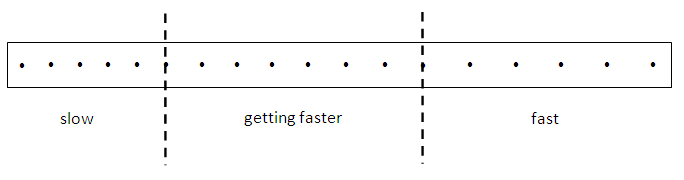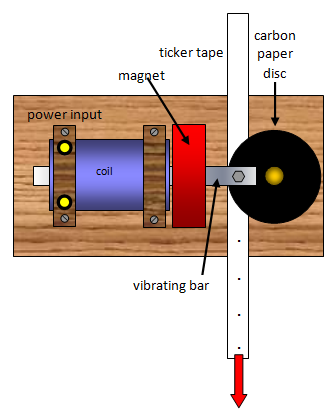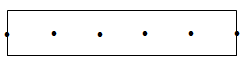# The ticker timer and speed

The ticker timer is simply a piece of apparatus that we use to measure time. When you work out the speed of an object you need to know how far it goes in a certain time.The distance is fairly easy to find, use a ruler, but if the object is moving quickly the time it takes will be hard to measure.The ticker timer makes dots on a paper tape every fiftieth of a second. So if a piece of tape is pulled through the timer for a second there will be 50 dots on it.

Since the spaces between the dots take such a short time (1/50 s) the ticker timer is a very useful instrument for measuring short intervals of time.

A piece of tape is shown in the diagram. You can see that the faster the tape is pulled through the timer the furthest apart the dots get. If the tape is pulled through at a steady speed the dots remain the same distance apart.

We have to be very careful here; as there is 1/50 s between dots we must count the number of SPACES and not the number of dots themselves.

To work out the velocity of the tape all we need to do is to measure the length of tape that goes through in the time given by the number of spaces.

If you have a length of tape with 50 spaces then it took 50/50 s or 1s to go through the timer. So if there are 5 spaces it took 5/50 = 1/10s.

Example problemWorking out the speed

The piece of tape in the diagram is 5 SPACES long.
Each space is 1/50 s so five spaces will be 5/50 s or 1/10s.
So the piece of tape went through the timer in 1/10s. The piece of tape is 7 cm long.

If 7cm of tape go through in 1/10 s then 70cm will go through in 1 s.
The speed is then 70 cm/s.

A VERSION IN WORD IS AVAILABLE ON THE SCHOOLPHYSICS USB